# Set Theory - Function (Mathematical)

A function in set theory is known as a mathematical function.

It is a relation between sets that associates:

• every element of the first set
• to exactly one element of the second set.

Duplicate ! Linear Algebra - Function (Set)

## Syntax

$$y = f(x)$$

## Physical Structure

A function is uniquely represented by the set of all pairs (ie a map) (x, f (x))

## Visualization

A function is represented graphically by the graph of the function

Recommended Pages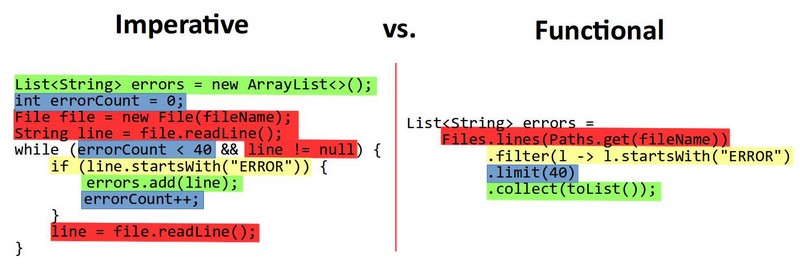Code - Functional programming (FP) - Collection Operations

Functional programming (FP) defines standard operations on collections. It is a declarative paradigm that treats computation as the evaluation of mathematical functions. Most of the operations, you perform...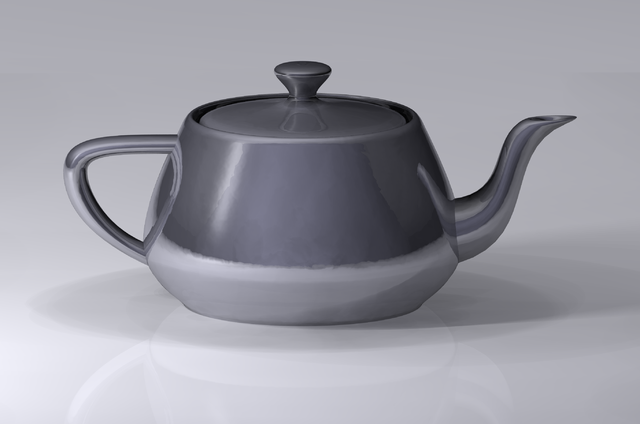Data Visualization - ( Chart | Graph | Graphic)

A chart, also called a graph (in mathematics for instance), is a graphical representation of data, in which the data is represented by symbols, such as: bars in a bar chart, lines in a line chart,...Language - Operator

An operator is a symbol (token) that compose an expression. It operates on values on its side (left and/or right). They represents a function but where the argument are on both side of it. This...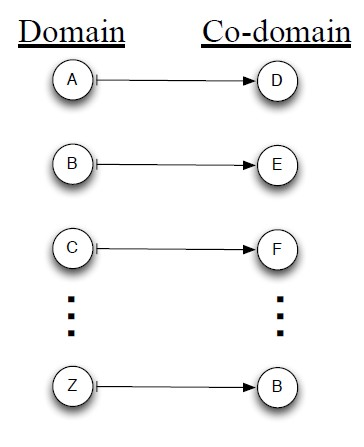Linear Algebra - Function (Set)

For each input element in a set , a function assigns a single output element from another set . is called the domain of the function is called the co-domain or in Mathese: The function...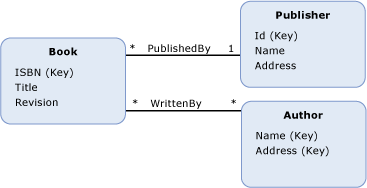Logical Data Modeling - Association

An association is a relationship between two or more entities. It's represented by a link in the data model. An association: answers to the question why two entity need to know one another. specifies...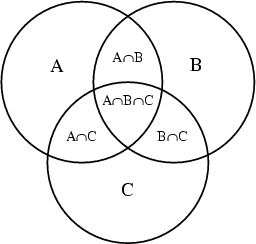Set - Graph of a mathematical function

graph of a mathematical function Graph of the Gaussian density function made with the World library ()...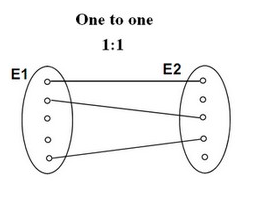Set Theory - Bijection function (One-to-one relationship)

A Bijection is a mathematical / set function between the elements of two sets (ie binary function) where each element of one set is paired with exactly one element of the other set, each element...Set Theory - Relationship

The relationships between set structure is: given by a mathematical function and is described by the set operators. You can visualize them with venn diagram.